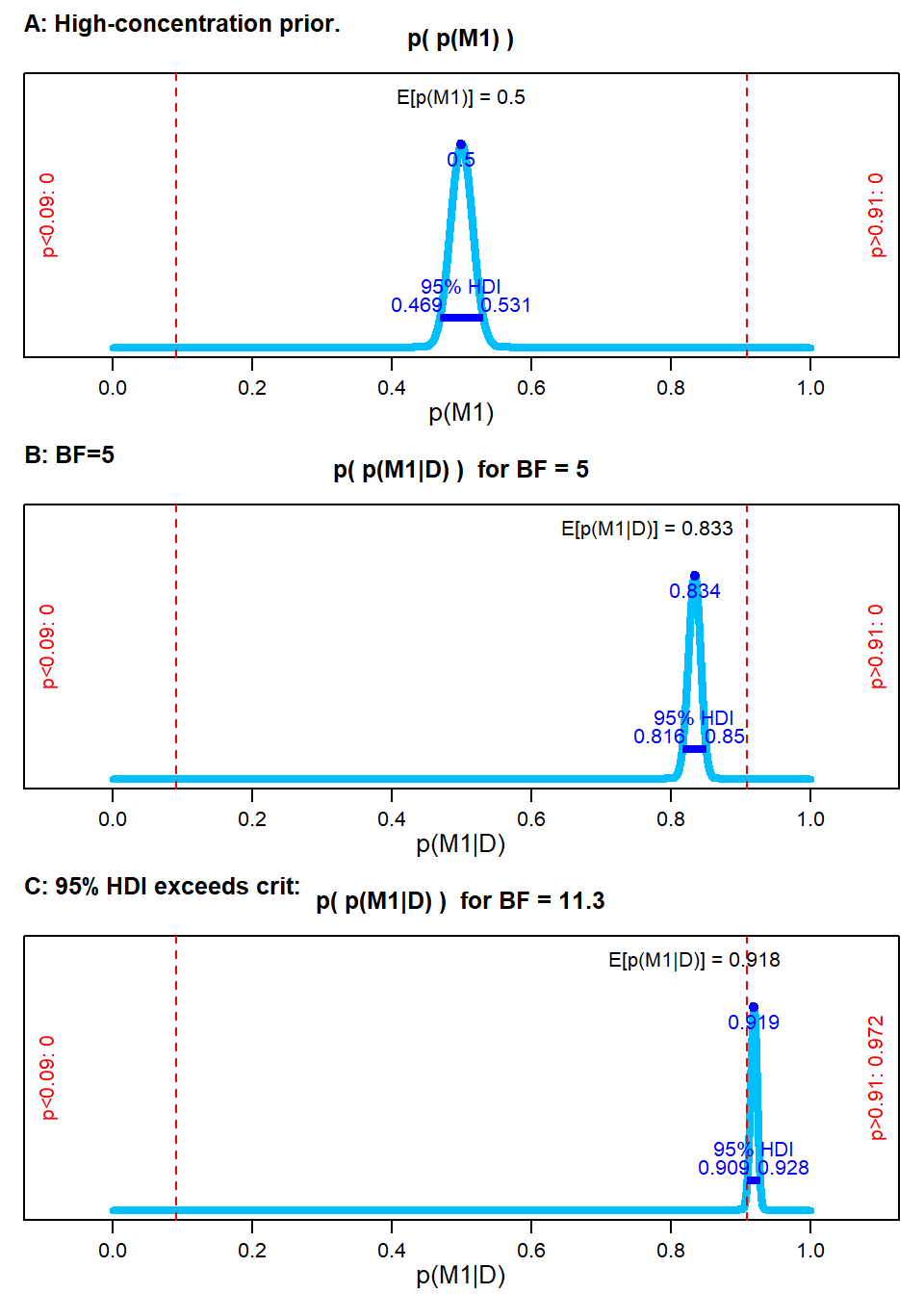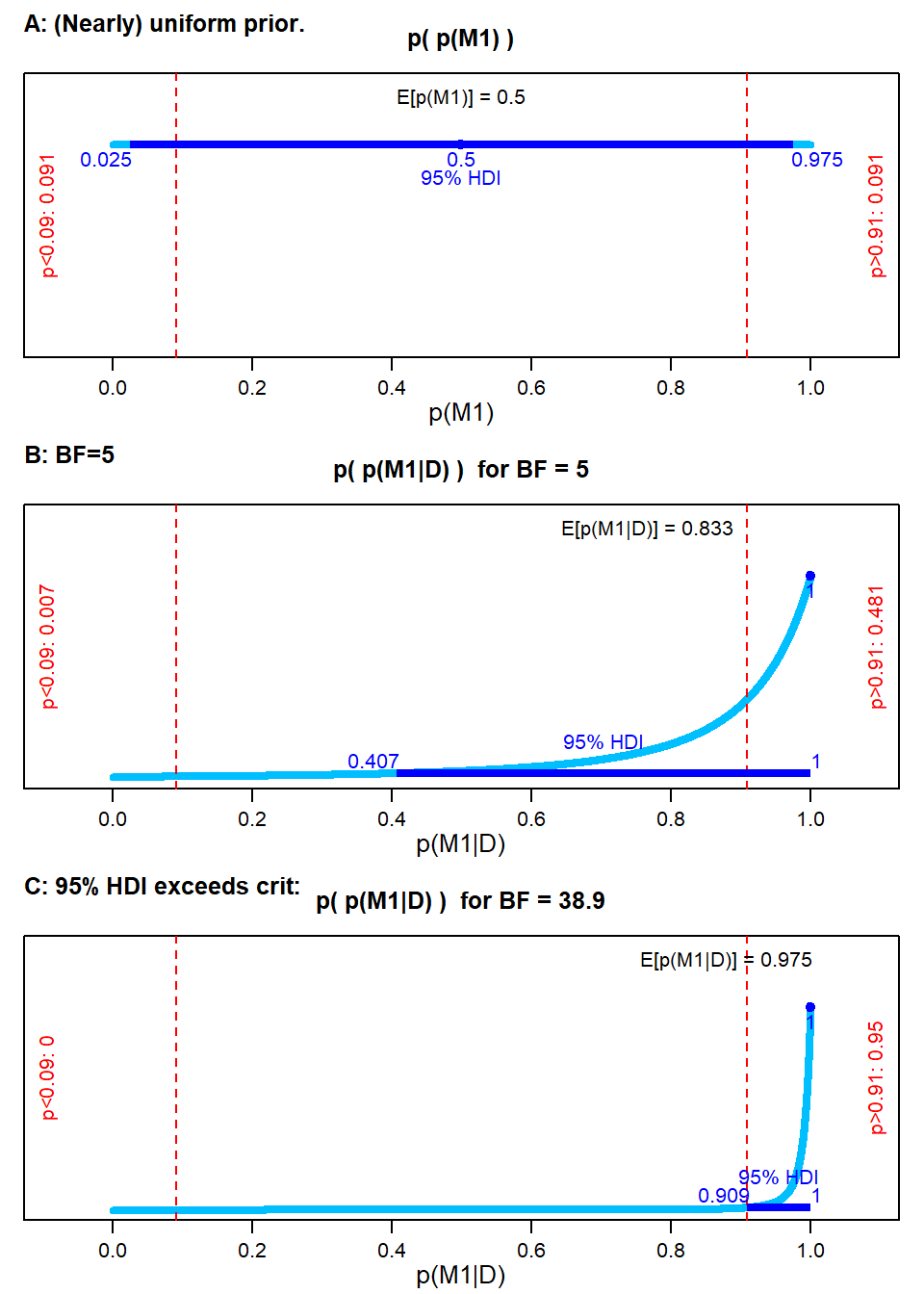## Friday, April 16, 2021

### Benchmark Bayes factors for uncertain prior model probability

I've posted a new manuscript titled "Uncertainty of prior and posterior model probability: Implications for interpreting Bayes factors." Here's a summary and examples to stimulate your interest.

Summary:

In most applications of Bayesian model comparison or Bayesian hypothesis testing, the results are reported in terms of the Bayes factor only, not in terms of the posterior probabilities of the models. Posterior model probabilities are not reported because researchers are reluctant to declare prior model probabilities, which in turn stems from uncertainty in the prior. Fortunately, Bayesian formalisms are designed to embrace prior uncertainty, not ignore it. This article provides

• novel formal derivations expressing the prior and posterior distribution of model probability
• a candidate decision rule that incorporates posterior uncertainty
• numerous illustrative examples
• benchmark BF’s using the uncertainty-based decision rule including benchmarks for a conventional uniform prior
• computational tools in R that are freely available at https://osf.io/36527/

I hope that this article provides both a conceptual framework and useful tools for better interpreting Bayes factors in all their many applications.

Examples:

Suppose you're doing a model comparison or hypothesis test, and you have a well-constructed Bayes factor of, say, BF=5. What do you conclude about the models or hypotheses? Your conclusion will depend on the posterior probabilities of the models, which in turn depend on the prior probabilities of the models. And what are the prior probabilities of the models? You're probably uncertain about the prior probabilities. Instead of pretending to have some specific point value for the prior model probabilities (as is usually done if it's done at all), we can represent the uncertainty as a distribution. The distribution of prior model probability becomes a distribution of posterior model probability, and we consider the entire distribution to decide about the models.

Notation: M1 is model 1, M2 is model 2. p(M1) is the prior probability of M1. BF is the Bayes factor for M1 relative to M2.

Figure 1, below, shows an example with a high-certainty (a.k.a., narrow, high-concentration) prior distribution at p(M1)=0.5. This prior distribution (see Panel A of Figure 1) represents a belief that the prior odds, p(M1)/p(M2), are almost certainly 50/50. When people assume any prior odds at all, this is the usual conventional for representing neutrality. Panel B shows the posterior distribution for BF=5 in favor of M1. Notice the probability of M1 has increased. Panel C shows the posterior distribution for BF=11.3, which is sufficient for the 95% HDI of the posterior distribution to exceed a decision criterion indicated by the vertical dashed line.Figure 1. A: High-certainty prior on p(M1). B: Posterior when BF=5. Posterior when BF=11.3.

Figure 2, by contrast, shows an example with a very uncertain (a.k.a., broad, low-concentration) prior distribution. This prior distribution (see Panel A of Figure 2) represents a much more typical state of prior knowledge, or at least is a much better representation of neutrality between models. Panel B shows the posterior distribution for BF=5. Notice it is very spread out. Panel C shows the posterior distribution for BF=38.9, which is sufficient for the 95% HDI of the posterior distribution to exceed a decision criterion (again indicated by the vertical dashed line). This BF might be treated as a benchmark when assuming a more realistic "neutral" prior for the model probabilities.Figure 2. A: Broad, uncertain prior on p(M1). B: Posterior when BF=5. C: Posterior when BF=38.9.

All the details are in the manuscript at https://osf.io/36527/. Please send me an email if you have comments!